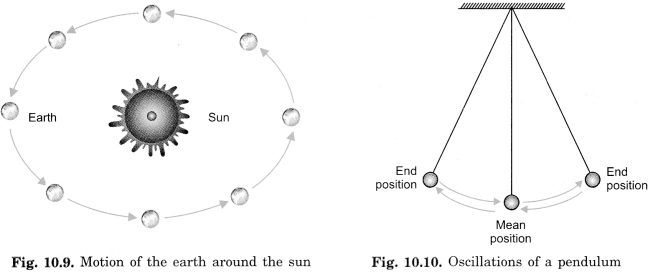NCERT Solutions for Class 6 Science Chapter 10 Motion and Measurement of Distances are part of NCERT Solutions for Class 6 Science. Here we have given NCERT Solutions for Class 6 Science Chapter 10 Motion and Measurement of Distances.

 Board CBSE Textbook NCERT Class Class 6 Subject Science Chapter Chapter 10 Chapter Name Motion and Measurement of Distances Number of Questions Solved 10 Category NCERT Solutions

## NCERT Solutions for Class 6 Science Chapter 10 Motion and Measurement of Distances

NCERT TEXTBOOK EXERCISES
(Page 106)

Question 1.
Give two examples each, of modes of transport used on land, water, and air.

1. Land – Bus, car, bicycle.
2. Water – Motor-boat, ship.
3. Air – Aeroplane, Helicopter.

Question 2.
Fill in the blanks:

1. One meter is ………………………………. cm.
2. Five kilometers is ………………………………. m.
3. The motion of a child on a swing is ………………………………..
4. The motion of the needle of a sewing machine is ………………………………..
5. The motion of the wheel of a bicycle is ………………………………..

1. 100
2. 5000
3. Periodic (oscillatory) motion
4. Periodic motion
5. Circular motion

Question 3.
Why can a pace or a footstep not be used as a standard unit of length?
A pace or a footstep cannot be used as a standard unit of length because it is different for different persons. Hence correct measurement cannot be made.

Question 4.
Arrange the following lengths in their increasing magnitude: 1 meter, 1 centimeter, 1 kilometer, 1 millimeter.
1 millimeter, 1 centimeter, 1 meter, 1 kilometer.

Question 5.
The height of a person is 1.65 m. Express it into cm and mm.

(1) 1 m = 100 cm.
∴ 1.65 = 165 cm.

(2) 1 cm = 10 mm
∴ 165 cm = 1650 mm
Hence the height of a person is 165 cm or 1650 mm.

Question 6.
The distance between Radha’s home and her school is 3250 m. Express this distance into km.
Given that,
Distance between Radha’s home and her school = 3250 m We have to find out distance in km.
We know that,
1000 m = 1 km
hence, 3250 m = $$\frac { 3250 }{ 1000 }$$ km = 3.250 km.

Question 7.
While measuring the length of a knitting needle, the reading of the scale at one end is 3.0 cm and at the other end is 33.1 cm. What is the length of the needle?
= 33. cm – 3.0 cm
= 30.1 cm.

Question 8.
Write the similarities and differences between the motion of a bicycle and a ceiling fan that has been switched on.

• Similarity: Both the wheels of a bicycle and a ceiling fan execute a circular motion on a fixed axis.
• Dissimilarity: A bicycle also executes a rectilinear motion while a fan does not execute such motion.

Question 9.
Why would you not like to use a measuring tape made of an elastic material like rubber to measure distance? What would be some of the problems you would meet in telling someone about a distance you measured with such a tape?
We could not use an elastic measuring tape to measure distance because elastic measuring tape cannot measure correct distance. Because if the elastic measuring tape is stretched more then the distance will be less and if it stretched less than the same distance would be more.
The problems we would meet in telling about the distance we measured with an elastic tape are that the distance would be incorrect and would be different every time measured.

Question 10.
Give two examples of periodic motion.